# Transient and Steady State Response in a Control System

When we study the analysis of the transient state and steady state response of control system it is very essential to know a few basic terms and these are described below.
Standard Input Signals : These are also known as test input signals. The input signal is very complex in nature, it is complex because it may be a combination of various other signals. Thus it is very difficult to analyze characteristic performance of any system by applying these signals. So we use test signals or standard input signals which are very easy to deal with. We can easily analyze the characteristic performance of any system more easily as compared to non standard input signals. Now there are various types of standard input signals and they are written below:

Unit Impulse Signal : In the time domain it is represented by ∂(t). The Laplace transformation of unit impulse function is 1 and the corresponding waveform associated with the unit impulse function is shown below.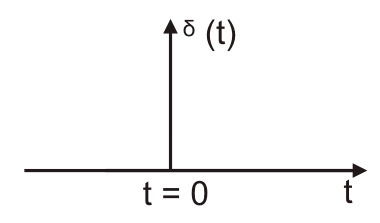Unit Step Signal : In the time domain it is represented by u (t). The Laplace transformation of unit step function is 1/s and the corresponding waveform associated with the unit step function is shown below.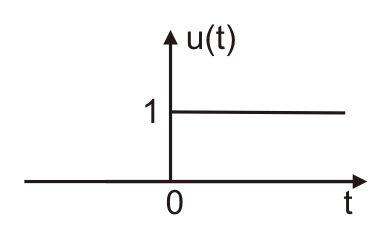Unit Ramp Signal : In the time domain it is represented by r (t). The Laplace transformation of unit ramp function is 1/s2 and the corresponding waveform associated with the unit ramp function is shown below.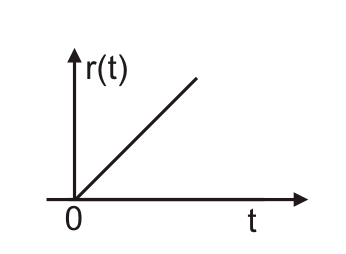Parabolic Type Signal : In the time domain it is represented by t2/2. The Laplace transformation of parabolic type of the function is 1/s3 and the corresponding waveform associated with the parabolic type of the function is shown below.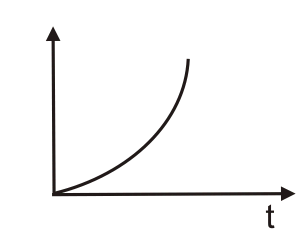Sinusoidal Type Signal : In the time domain it is represented by sin (ωt).The Laplace transformation of sinusoidal type of the function is ω / (s2 + ω2) and the corresponding waveform associated with the sinusoidal type of the function is shown below.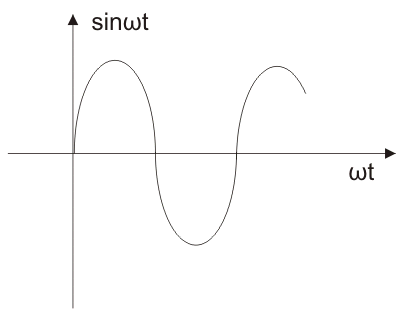Cosine Type of Signal : In the time domain it is represented by cos (ωt). The Laplace transformation of the cosine type of the function is ω/ (s2 + ω2) and the corresponding waveform associated with the cosine type of the function is shown below,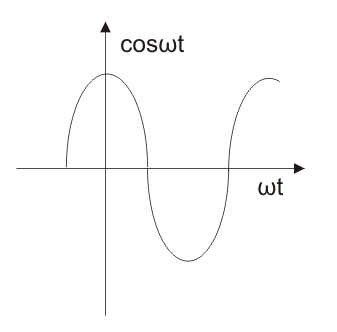Now are in a position to describe the two types of responses which are a function of time.

## Transient Response of Control System

As the name suggests transient response of control system means changing so, this occurs mainly after two conditions and these two conditions are written as follows-

• Condition one : Just after switching ‘on’ the system that means at the time of application of an input signal to the system.
• Condition second : Just after any abnormal conditions. Abnormal conditions may include sudden change in the load, short circuiting etc.

## Steady State Response of Control System

Steady state occurs after the system becomes settled and at the steady system starts working normally. Steady state response of control system is a function of input signal and it is also called as forced response.

Now the transient state response of control system gives a clear description of how the system functions during transient state and steady state response of control system gives a clear description of how the system functions during steady state. Therefore the time analysis of both states is very essential. We will separately analyze both the types of responses. Let us first analyze the transient response. In order to analyze the transient response, we have some time specifications and they are written as follows:
Delay Time : This time is represented by td. The time required by the response to reach fifty percent of the final value for the first time, this time is known as delay time. Delay time is clearly shown in the time response specification curve.

Rise Time: This time is represented by tr, and can be calculated using the rise time formula. We define rise time in two cases:

1. In case of under damped systems where the value of ζ is less than one, in this case rise time is defined as the time required by the response to reach from zero value to hundred percent value of final value.
2. In case of over damped systems where the value of ζ is greater than one, in this case rise time is defined as the time required by the response to reach from ten percent value to ninety percent value of final value.

Peak Time: This time is represented by tp. The time required by the response to reach the peak value for the first time, this time is known as peak time. Peak time is clearly shown in the time response specification curve.

Settling Time: This time is represented by ts, and can be calculated using the settling time formula. The time required by the response to reach and within the specified range of about (two percent to five percent) of its final value for the first time, this time is known as settling time. Settling time is clearly shown in the time response specification curve.

Maximum Overshoot: It is expressed (in general) in percentage of the steady state value and it is defined as the maximum positive deviation of the response from its desired value. Here desired value is steady state value.
Steady state error: Defined as the difference between the actual output and the desired output as time tends to infinity.Now we are in position we to do a time response analysis of a first order system.

## Transient State and Steady State Response of First Order Control System

Let us consider the block diagram of the first order system.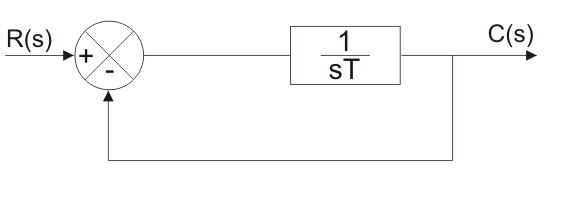From this block diagram we can find overall transfer function which is linear in nature. The transfer function of the first order system is 1/((sT+1)). We are going to analyze the steady state and transient response of control system for the following standard signal.

1. Unit impulse.
2. Unit step.
3. Unit ramp.

Unit impulse response : We have Laplace transform of the unit impulse is 1. Now let us give this standard input to a first order system, we have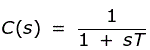Now taking the inverse Laplace transform of the above equation, we have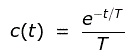It is clear that the steady state response of control system depends only on the time constant ‘T’ and it is decaying in nature.
Unit Step Response : We have Laplace transform of the unit impulse is 1/s. Now let us give this standard input to first order system, we have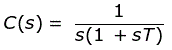With the help of partial fraction, taking the inverse Laplace transform of the above equation, we have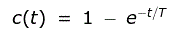It is clear that the time response depends only on the time constant ‘T’. In this case the steady state error is zero by putting the limit t is tending to zero.
Unit Ramp Response : We have Laplace transform of the unit impulse is 1/s2. Now let us give this standard input to first order system, we have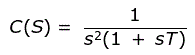With the help of partial fraction, taking the inverse Laplace transform of the above equation we have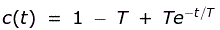On plotting the exponential function of time we have ‘T’ by putting the limit t is tending to zero.

## Transient State and Steady State Response of Second Order Control System

Let us consider the block diagram of the second order system.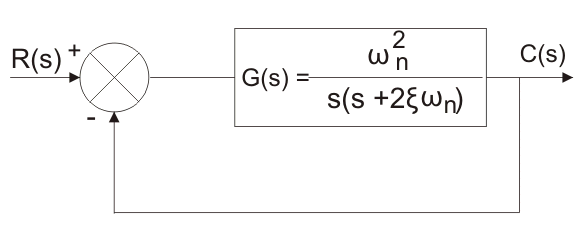From this block diagram we can find overall transfer function which is nonlinear in nature. The transfer function of the second order system is (ω2) / {s (s + 2ζω )}. We are going to analyze the transient state response of control system for the following standard signal.

Unit Impulse Response : We have Laplace transform of the unit impulse is 1. Now let us give this standard input to second order system, we have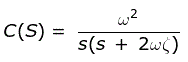Where, ω is natural frequency in rad/sec and ζ is damping ratio.
Unit Step Response : We have Laplace transform of the unit impulse is 1/s. Now let us give this standard input to first order system, we haveNow we will see the effect of different values of ζ on the response. We have three types of systems on the basis of different values of ζ.

1. Under Damped System : A system is said to be under damped system when the value of ζ is less than one. In this case roots are complex in nature and the real parts are always negative. System is asymptotically stable. Rise time is lesser than the other system with the presence of finite overshoot.
2. Critically Damped System : A system is said to be critically damped system when the value of ζ is one. In this case roots are real in nature and the real parts are always repetitive in nature. System is asymptotically stable. Rise time is less in this system and there is no presence of finite overshoot.
3. Over Damped System : A system is said to be over damped system when the value of ζ is greater than one. In this case roots are real and distinct in nature and the real parts are always negative. System is asymptotically stable. Rise time is greater than the other system and there is no presence of finite overshoot.
4. Sustained Oscillations : A system is said to be sustain damped system when the value of zeta is zero. No damping occurs in this case.

Now let us derive the expressions for rise time, peak time, maximum overshoot, settling time and steady state error with a unit step input for second order system.
Rise Time : In order to derive the expression for the rise time we have to equate the expression for c(t) = 1. From the above we haveOn solving above equation we have expression for rise time equal to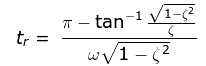Peak Time : On differentiating the expression of c(t) we can obtain the expression for peak time. dc(t)/ dt = 0 we have expression for peak time,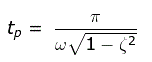Maximum Overshoot : Now it is clear from the figure that the maximum overshoot will occur at peak time tp hence on putting the value of peak time we will get maximum overshoot as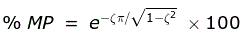Settling Time : Settling time is given by the expression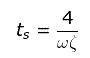Steady State Error : The steady state error is diffrerence between the actual output and the desired output hence at time tending to infinity the steady state error is zero.

Want To Learn Faster? 🎓
Get electrical articles delivered to your inbox every week.
No credit card required—it’s 100% free.